# Polyphase Power Systems

## AC Electric Circuits

• #### Question 1

Explain the difference between a balanced polyphase system and an unbalanced polyphase system. What conditions typically cause a polyphase system to become unbalanced?

• #### Question 2

What is polyphase electric power, and how does it differ from single-phase power?

• #### Question 3

All large electric power generating systems in the world today produce three phase power, rather than single phase power. Explain why this is so, in terms of advantages of distribution and use.

• #### Question 4

How is polyphase (especially three-phase) electric power generated? Single-phase power is easy to understand, but how do we create three-phase AC voltage?

• #### Question 5

Suppose a set of three neon light bulbs were connected to an alternator with three sets of windings labeled A, B, and C: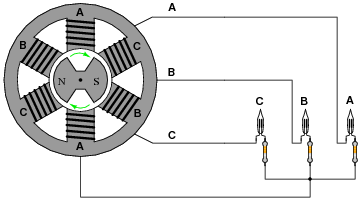The schematic diagram for this alternator/lamp system is as follows: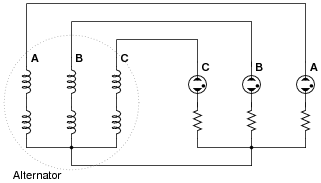If the alternator spins fast enough (clockwise, as shown), the AC voltage induced in its windings will be enough to cause the neon lamps to “blink” (neon bulbs have very fast reaction times and thus cannot maintain a glow for very long without current, unlike incandescent lamps which operate on the principle of a glowing-hot metal filament). Most likely this blinking will be too fast to discern with the naked eye.

However, if we were to video record the blinking and play back the recording at a slow speed, we should be able to see the sequence of light flashes. Determine the apparent “direction” of the lamps’ blinking (from right-to-left or from left-to-right), and relate that sequence to the voltage peaks of each alternator coil pair.

• #### Question 6

What is meant by the term phase rotation sequence, in a polyphase electrical system?

• #### Question 7

This is a schematic diagram of a Y-connected three-phase generator (with the rotor winding shown):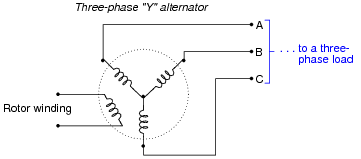How much AC voltage will appear between any two of the lines (VAB, VBC, or VAC) if each stator coil inside the alternator outputs 277 volts? Draw a phasor diagram showing how the phase (winding) and line voltages relate.

• #### Question 8

This is a schematic diagram of a Delta-connected three-phase generator (with the rotor winding shown):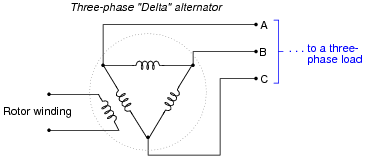How much AC current will each of the lines (IA, IB, or IC) conduct to a load (not shown) if each stator coil inside the alternator outputs 17 amps of current?

• #### Question 9

Suppose the electrical power supplied to a commercial building is labeled as “208/120 volt”. What does this label mean, exactly? Relate this description to a schematic diagram.

• #### Question 10

Suppose you are working on the power wiring inside of a home, and are wondering whether or not the home is supplied with 3-phase or single-phase power. You do not have a voltmeter available to measure voltage, but you do have plenty of light bulbs, switches, wires, and other standard residential wiring components available for use.

The two possibilities for this home’s power source are shown here, the coils representing secondary windings of the utility power transformer: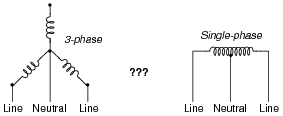An experienced electrician suggests you build the following circuit to test whether or not the home’s power is supplied by a 3-phase source or a single-phase source: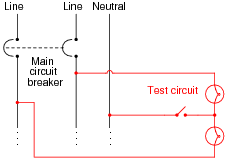The electrician tells you to open and close the switch, and observe the brightness of the light bulbs. This will indicate whether or not the system is 3-phase.

Explain how this circuit works. What sort of light bulb behavior would indicate a 3-phase source? What sort of light bulb behavior would indicate a single-phase source?

• #### Question 11

This Delta-connected three-phase power source provides three different voltage levels: 120 V, 208 V, and 240 V. Determine which points of connection provide these voltages:• #### Question 12

The line voltage to this three-phase load is 480 volts. How much power (total) is dissipated by the load? How much current is there in each line supplying the load?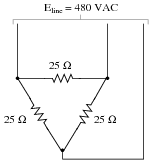One more question: write an equation for calculating power in a balanced, three-phase circuit, given line voltage and line current only.

• #### Question 13

A balanced, three-phase power system has a line voltage of 13.8 kV volts and a line current of 150 amps. How much power is being delivered to the load (assuming a power factor of 1)?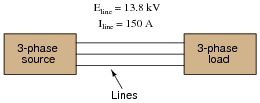A 13.8 kV single-phase system could be designed to provide the same amount of power to a load, but it would require heavier-gauge (more expensive!) conductors. Determine the extra percentage of expense in wire cost (based on the weight of the wires) resulting from the use of single-phase instead of three-phase.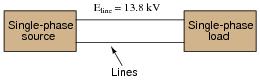• #### Question 14

Calculate the full-load line current for a three-phase motor, given a horsepower rating of 150 HP, an efficiency of 93%, and a line voltage of 480 volts. Assume a power factor of 0.90 for full-load conditions.